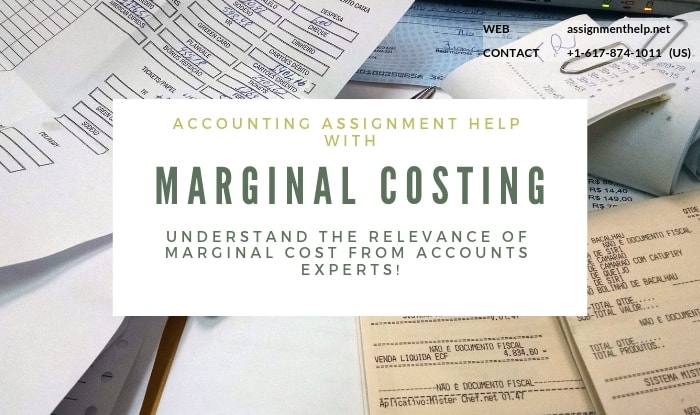# Marginal Costing Assignment Help

## Marginal costing and Break even analysis

### 1.1 Meaning of Marginal Costing

Marginal cost means cost of producing additional unit. In the other words we can say change in total cost due to change in output. This happens because variable cost changes with level of output whereas fixed cost remains same given no change in capacity utilisation rate. Accordingly, cost of producing one additional unit is generally the variable cost for one unit. However this is theoretical proposition. In practice, production changes from one capacity level to another. So marginal cost may be defined as the sum of Variable cost per unit and incremental fixed cost per unit.

### 1.2 Relevance of Marginal Cost

Now the question arises why should we at all bother about the marginal cost? This is because for profit maximization marginal cost should always be lower than marginal revenue. Otherwise the firm would not be able to maximise its profit.If the selling price per unit of the product let’s say is Rs 400, Variable cost is Rs 320 per unit and total fixed cost is Rs.360,000 then, The profit margin is Rs 20 per unit, then the total profit earned for 6000 units is Rs 120,000.

Now suppose If the factory produces 7000 units assuming there would no change in capacity and the additional units(1000) can be sold only at discount, the marginal revenue is Rs 360 (Rs 400 – 10% of Rs 400 ). It gives a profit of Rs 40 per unit.

Marginal Revenue = Rs 360

Marginal Cost = Rs 320

Profit per unit of additional output = 40

Additional Profit: Rs 40 x 1000 = Rs 40,000. Thus additional production works out to be profitable.

## Marginal Costing Assignment Help By Online Tutoring and Guided Sessions from AssignmentHelp.Net